# RIGAKU JOURNAL: PDF ANALYSIS USING X-RAY TOTAL SCATTERING - THEORY AND APPLICATION EXAMPLES

### Masatsugu Yoshimoto and Yuji Shiramata

In recent years, pair distribution function (PDF) analysis has been used to characterize material structure in a wide research field. H. Kim reported the reduction mechanism in the reversible hydrogen storage capacity of V1xTix alloys. B. Li et al., reported the mechanisms of the thermoelectric effect and the phase transition to superionic conductor of AgCrSe, which is known to be a thermoelectric material at high temperature, by X-ray and neutron total scattering, and inelastic neutron scattering. K. Ohara et al. reported on the process of crystallization of Li7P3S11, the most important material for all-solid-state Li ion batteries, carried out by a time-resolved PDF measurement at a synchrotron source. This measurement technique can be applied to investigate structural changes in real time.

In 1927, the basic theory of PDF analysis was first reported by Zernike–Prins. They reported that the structure factor observed from an X-ray scattering profile directly corresponds to the PDF. PDF analysis mainly provides local structural information, such as coordination number and average interatomic distances. PDF analysis can be applied to any sample conditions (i.e., crystalline solid, amorphous solid and fluid); however, this analysis has been mainly used for structural analysis of amorphous solids and liquid. Recently, PDF analysis has also been used to characterize the local structure of crystalline materials.

Figure 1 shows the X-ray scattering profile of crystalline and amorphous carbon. The XRD profile of amorphous carbon has broad peaks compared to crystalline carbon; however, the peak positions are almost the same for both samples. These results indicate
that amorphous carbon lacks a long-range ordered structure compared to crystalline carbon.

Figure 2 shows the radial distribution function (RDF) against interatomic distances. Each peak position in the RDF corresponds to 1st (red dotted line), 2nd (blue dotted line), 3rd (green dotted line) . . . neighbor distances between carbon atoms shown in Fig. 3.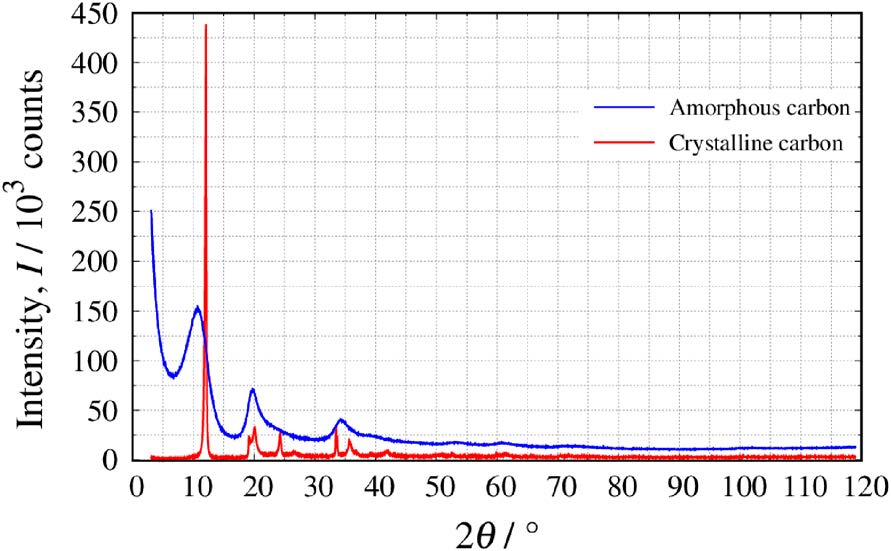Fig. 1. X-ray scattering profiles of crystalline carbon and amorphous carbon.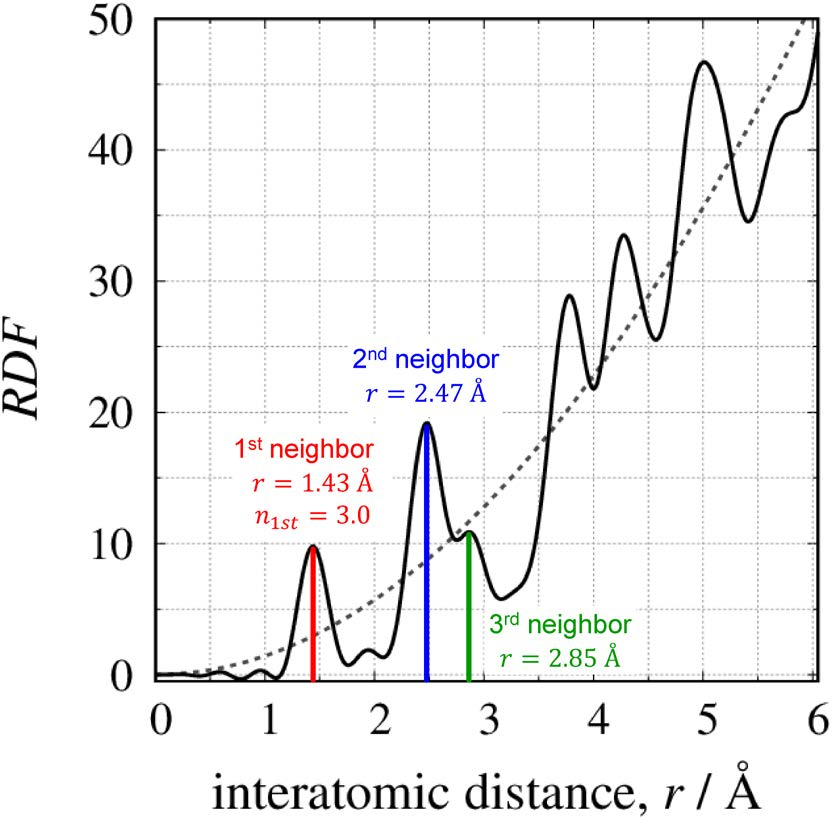Fig. 2. Radial distribution function (RDF) obtained from X-ray scattering profile of amorphous carbon.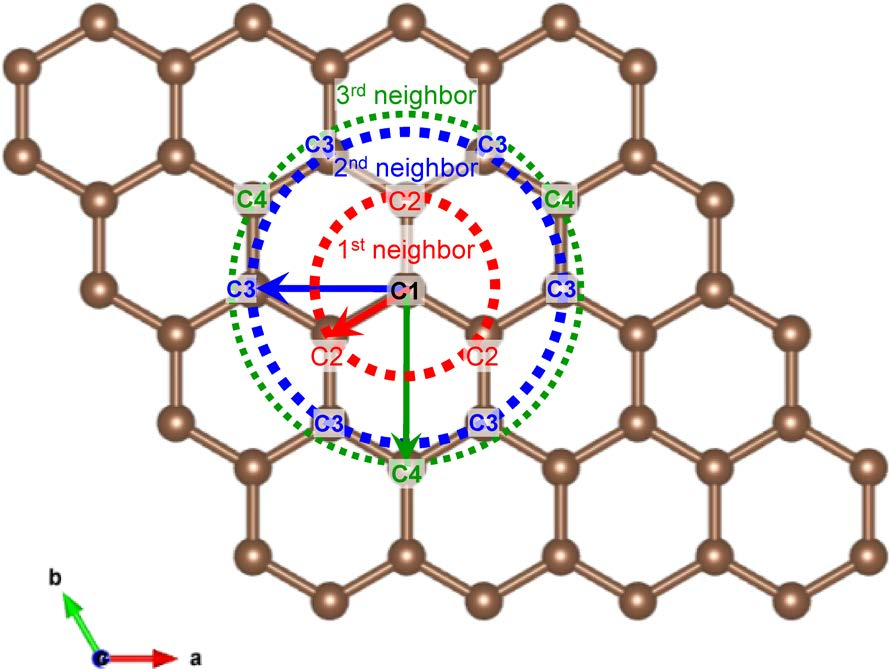Fig. 3. Two-dimensional structural model of the carbon crystal.

The aim of this paper is to introduce the theoretical background of PDF, measurement systems, and application examples. (This data courtesy of Assistant Prof. Kubota, Komaba Group, Tokyo University of Science)

### 2. Theory

The observed intensity of X-rays, Iobs, scattered by a material mainly includes three components: coherent scattering intensity, Icoh, incoherent scattering intensity,
Iincoh (i.e., Compton scattering) and X-ray fluorescence intensity, IXRF.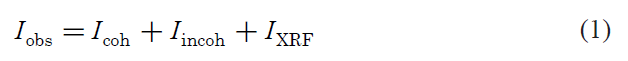PDF analysis requires only the coherent scattering intensity, Icoh; therefore, incoherent scattering Iincoh is eliminated based on theoretical calculations, and X-ray fluorescence IXRF can be removed using a discriminator in the detector and/or a crystal monochromator. In the
case of isotropic materials, Icoh is expressed by equation 2. Q(Å－1) in equation 2 is a scattering vector, defined by equation 3.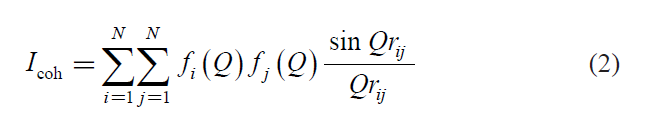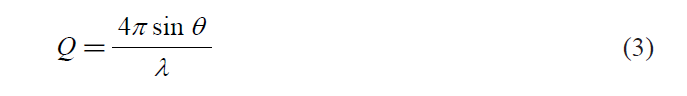where N is the number of atoms in the system, ƒi (Q) and ƒj (Q) are the atomic scattering factors of the i-th and j-th atom, respectively, and rij is the distance between the i-th and j-th atoms. This equation is called the Debye scattering formula(5).

Defining ρ(r) as the atomic density at distance r from an atom, the number of atoms that exist in a spherical
shell between radius r and r＋dr is 4πr2 ρ(r)dr (4πr2 dr is the volume of spherical shell).
If, for simplicity, we consider a material consisting of a single element, equation 2 can also be rewritten as shown in equation 4(6)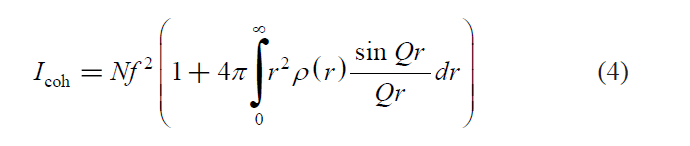Next, equation 4 can be rewritten to determine the deviation from average density, ρ0, in the system.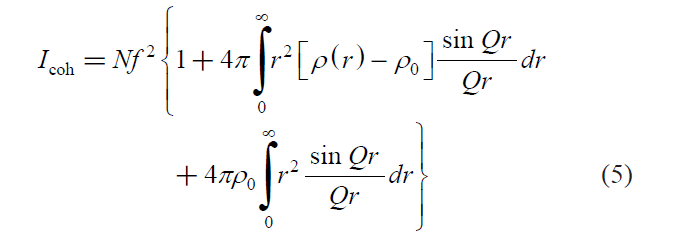In equation 5, the third term contributes to profiles at very small Q because this term expresses the scattering from the uniform atomic distribution of the entire system. In general PDF analysis, the third term can be treated as zero; therefore, equation 5 can be rewritten as follows(6).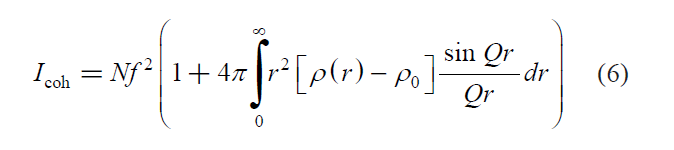Structure factor, S(Q), is obtained from the observed coherent scattering, Icoh.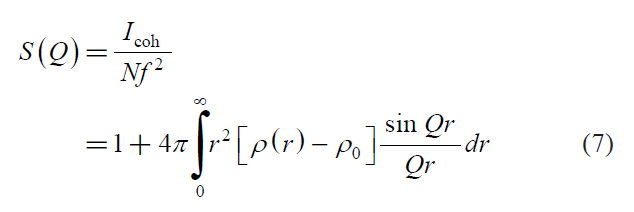S(Q) is expressed by the Fourier transformation of the local density deviation from its average density in the system, ρ0. Figure 4 shows the X-ray scattering profile and S(Q) for silica (SiO2) glass, using AgKα radiation (λ＝0.561 Å, Qmax＝22 Å－1).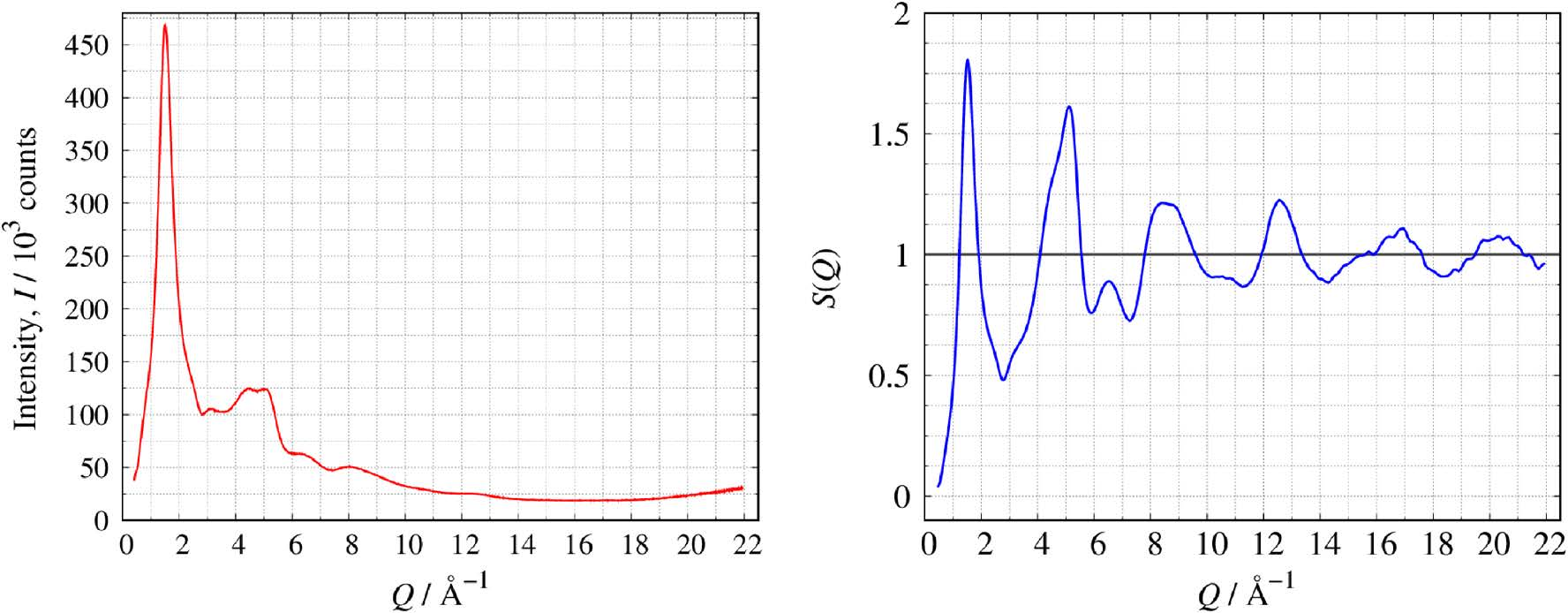Fig. 4. X-ray scattering profile (left) of SiO2 glass and derived S(Q) (right).

Equation 8 is expressed multiplying both sides of equation 7 by Q: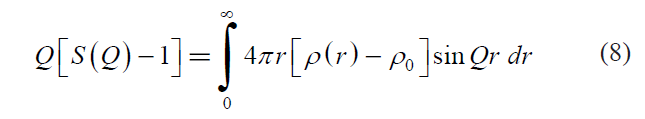By applying a Fourier transformation to equation 8 with Q, the following equation is derived: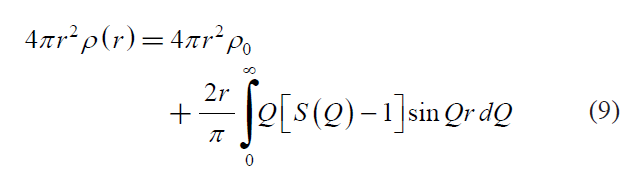where, 4πr2 ρ(r)N(r) is called the radial distribution function (RDF)(7).
The atomic pair distribution function g(r) is defined by the following equation: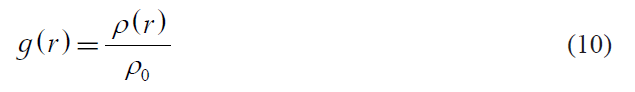By combining equations 9 and 10, g(r) can be calculated from the observed structure factor(7):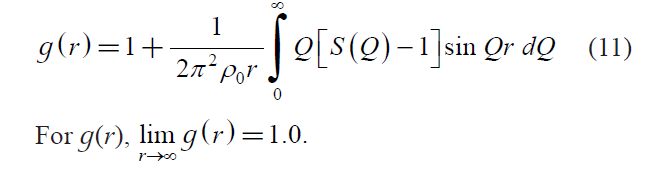To characterize crystalline structures, a function called the reduced atomic pair distribution function G(r) is generally used, which is related to g(r) by the following equation(7):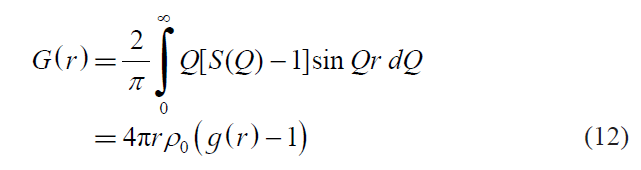It is easy to convert among N(r), g(r), and G(r).

Figure 5 illustrates N(r), g(r), and G(r) of silica (SiO2) glass.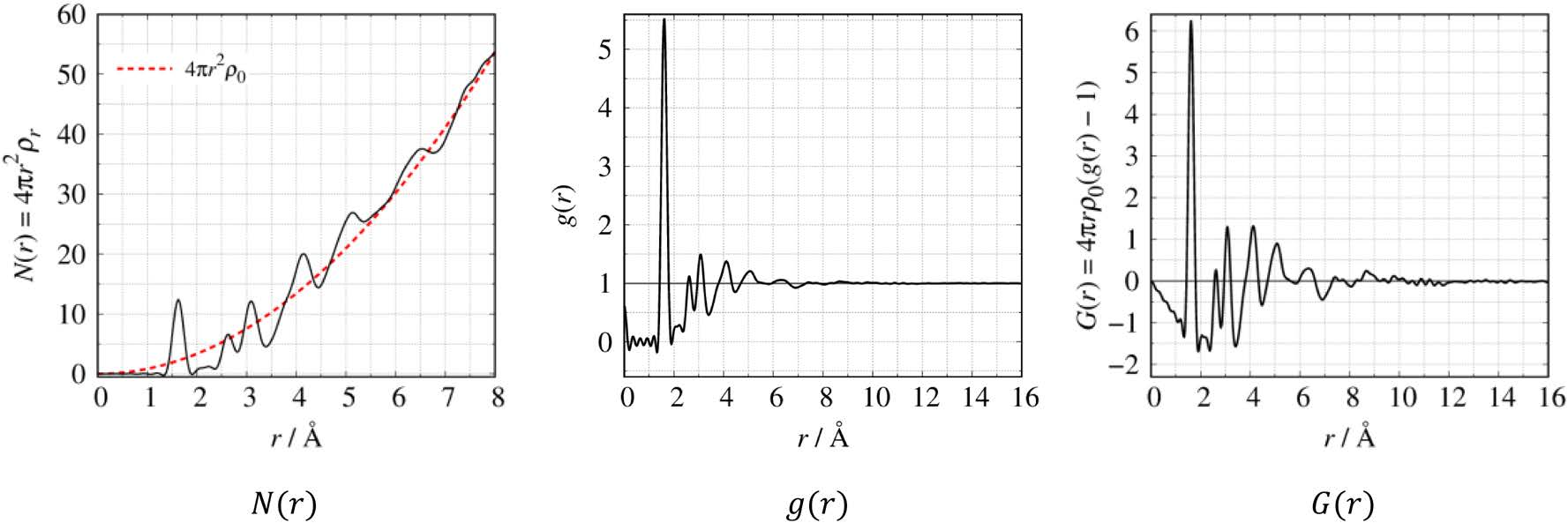Fig. 5. N(r), g(r), and G(r) of SiO2 glass.

N(r) shows an upward-sloping pattern, oscillating along the average density curve, 4πr2 ρ0 (red dotted curve). The RDF demonstrates that the coordination number increases with increasing distance r, proportional to the shell volume 4πr2dr.
The coordination number of certain neighbor distances can be calculated by the peak area of N(r), e.g., by using Gaussian fitting.

On the other hand, g(r), which is normalized by the average density in the system, oscillates around 1 (see equation 10). A large peak indicates that local atomic density is higher than the average and a deep valley indicates that local atomic density is lower than the average. At a distance far from the central atom, the local atomic density is close to the average, so the g(r) value is close to 1, as shown in Fig. 5. For the same sample, G(r) is enhanced at long distances compared to g(r). Therefore, it is preferred to investigate the local structure of crystalline materials along a medium distance range.
As described above, it is recommended to use the distribution function N(r), g(r), or G(r) that is best suited for the purpose of the analysis.

### 3. Measurement

#### 3.1. X-ray Sources

PDF analysis requires profiles with a high Q range. Therefore profiles should be measured to as high an angle (2θ≈160°) as possible using a short-wavelength X-ray source such as AgKα (λ＝0.561 Å, Qmax≈22 Å－1) or MoKα radiation (λ＝0.711 Å, Qmax≈17 Å－1). Next, the resolution in real space, Δr, can be estimated by the following equation.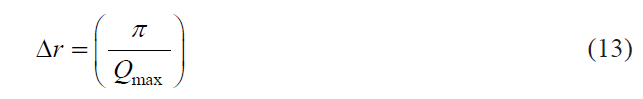Equation 13 indicates that Δr decreases with increasing Qmax value. In other words, a low Δr value means high real-space resolution. A PDF with high real-space resolution can be obtained from S(Q) by including the high Q region. Additionally, termination errors are an inescapable problem with Fourier transformations because the observed S(Q) has a finite range. S(Q) should be measured including the high Q region in order to reduce termination errors.

Figure 6 shows the X-ray scattering (left) and RDF profiles (right) for silica glass (SiO2) measured by X-ray sources with different wavelengths.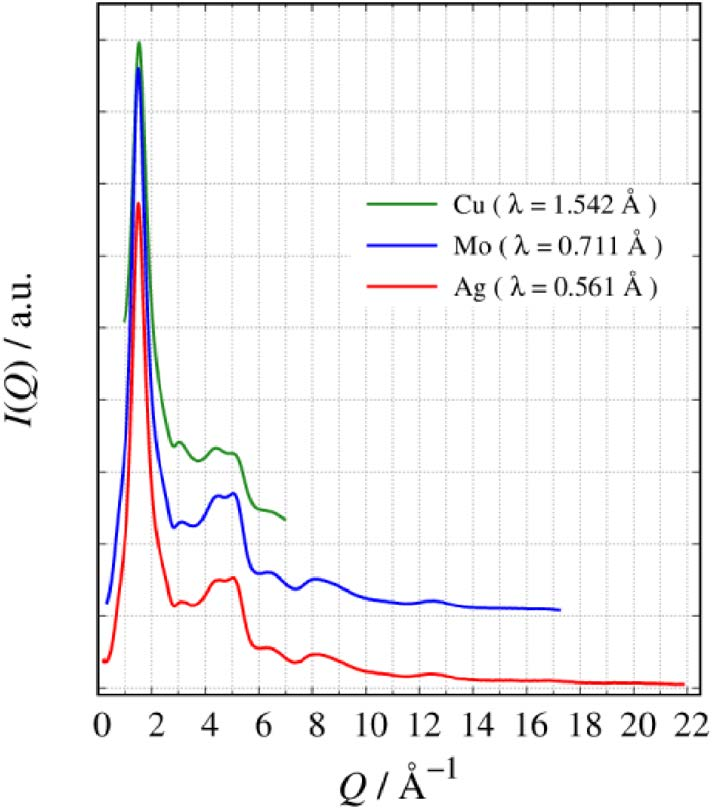Fig.6. X-ray scattering profiles of SiO2 glass obtained using CuKα, MoKα, and AgKα radiation (after offset processing.)

The S(Q) with high Q range can be obtained using a short wavelength (i.e. AgKα＜MoKα＜CuKα). Figure 7(a) shows G(r) measured by AgKα, MoKα and CuKα. Figure 7(b)
shows the SiO4 tetrahedral unit.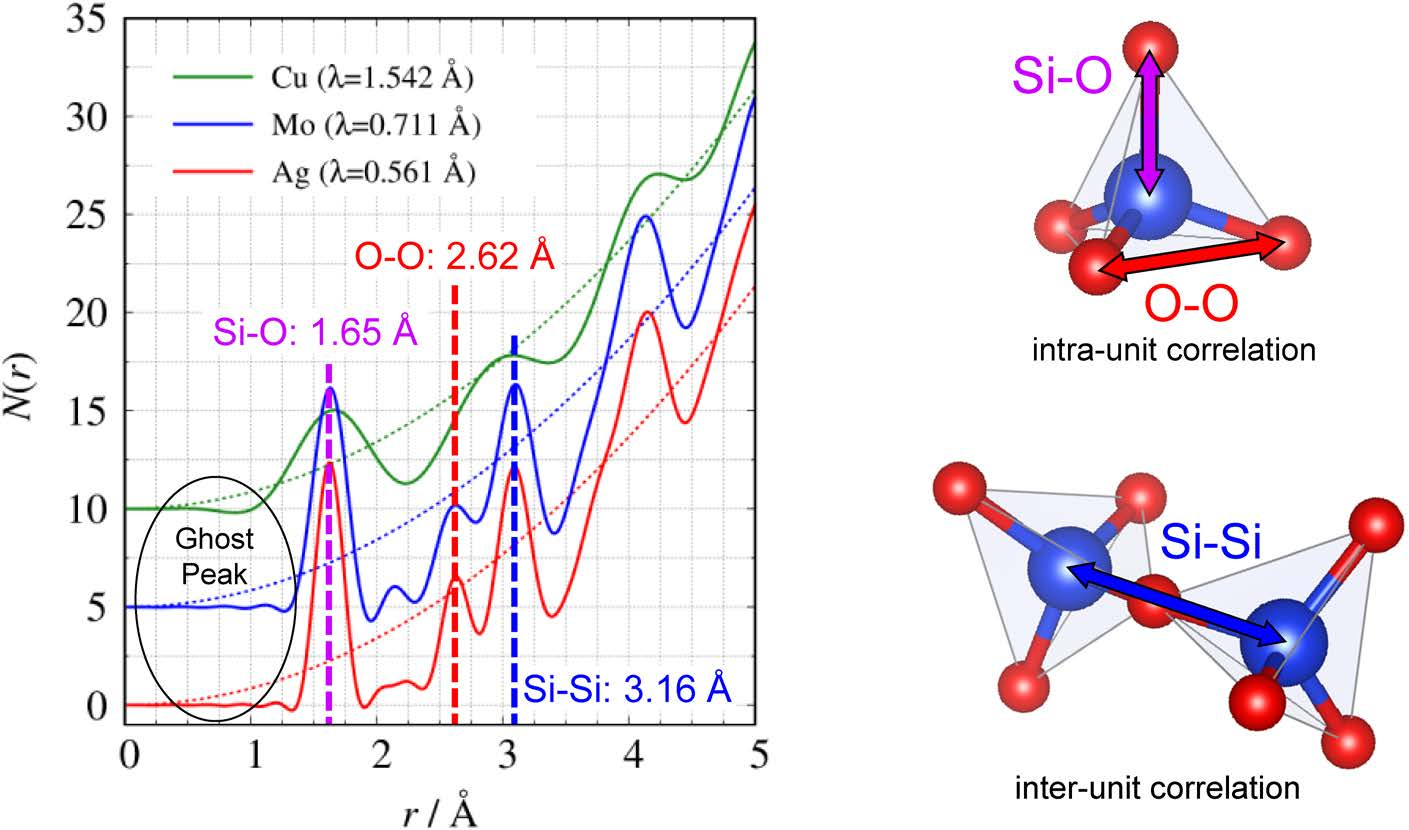Fig. 7. Radial distribution function SiO2 glass by CuKα (green), MoKα (blue) and AgKα (red) after offset processing. Three dash lines
indicate 4πr2ρ0 curve (left). Intra and inter correlations of SiO4 tetrahedral unit (right).

The RDFs measured by AgKα and MoKα have two peaks near r＝2.62, 3.16 Å, corresponding to O–O distances within a tetrahedral unit and Si–Si distances between tetrahedral units, respectively. However, the RDF measured by CuKα radiation cannot resolve these distances because of low resolution in real-space. CuKα radiation should not be selected for PDF analysis with high resolution in real-space.

For a long time, PDF analysis was thought to require a synchrotron source, because these sources can measure S(Q) with a wide Q range. However, nowadays, a laboratory diffractometer can measure S(Q) as well as a synchrotron source. Figure 8 shows S(Q) and g(r) of silica glass measured by a SmartLab (λ＝0.561 Å), which is a laboratory X-ray diffractometer, and those by a synchrotron source (E＝61 keV, λ＝0.203 Å). The peak positions and area of g(r) from the SmartLab show good agreement with that from the synchrotron source.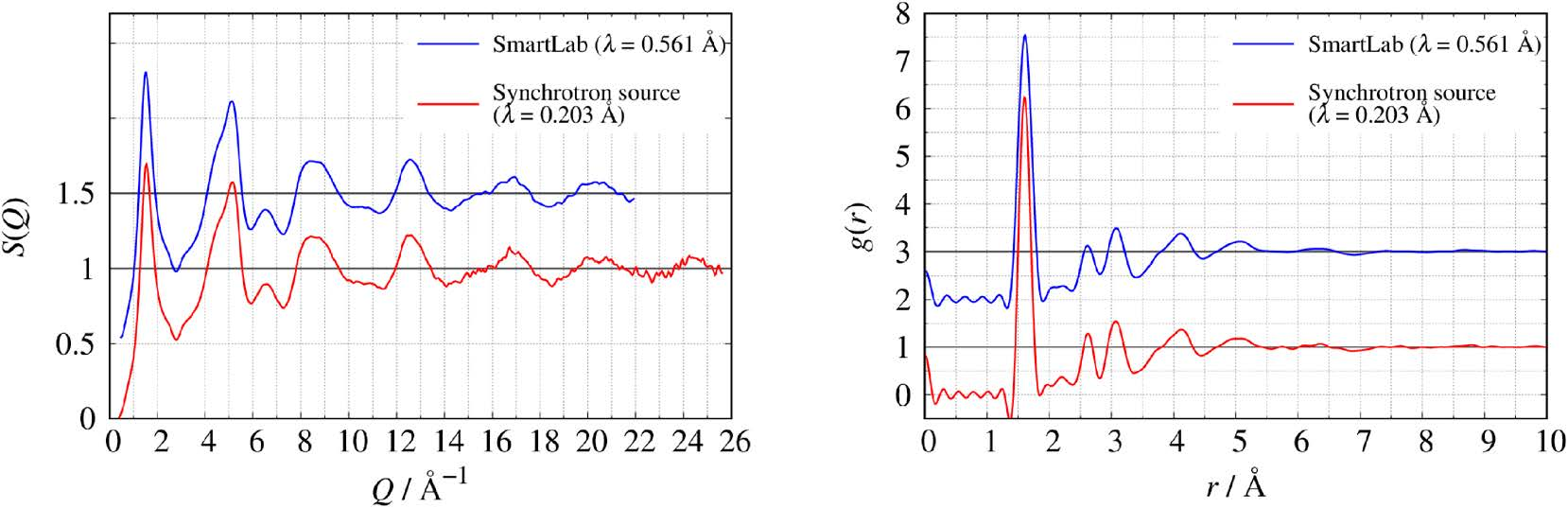Fig.8. S(Q) (left) and g(r) (right) of SiO2 glass obtained using synchrotron source and laboratory X-ray diffractometer SmartLab (after
offset processing).

#### 3.2. Experimental geometry

The SmartLab enables the use of both transmission and reflection geometry. In the former case, a container filled with liquid and powder samples can be measured. Cylindrical geometry, also known as Debye–Sherrer geometry, is the most appropriate for PDF analysis because the volume of X-ray irradiation is kept at the same value at any detector position. When a capillary tube is used as the sample container, background scattered from the container must be at a low level compared to the sample profile. A problem with reflection geometry (e.g., Bragg–Brentano geometry) is the large X-ray penetration depth into the sample surface. This method is generally appropriate for the measurement of bulk or powder samples filled in a punched-out sample holder.

#### 3.3. Optical devices and detectors

The SmartLab (show in Fig. 9) employs a high-power rotating anode capable of applying up to 60 kV-100 mA (6.0 kW) for an Ag target and up to 60 kV-150 mA (9.0 kW) for a Mo target. Therefore, the measurement intensity is higher using a high-power rotating anode than a sealed-tube (2.16 kW for Ag and 3.0 kW for Mo).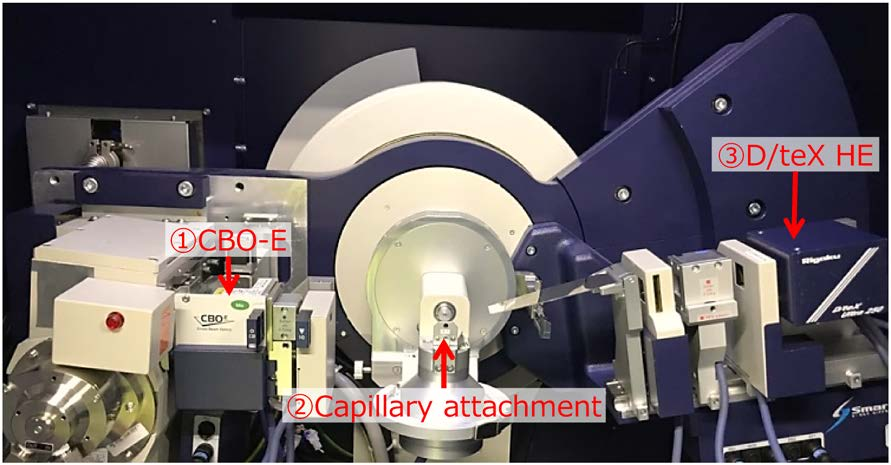Fig.9. Goniometer of SmartLab.

The SmartLab is designed to easily generate a convergent or parallel beam using multilayer mirrors, which can also provide monochromatic X-rays (i.e., MoKα or AgKα radiation). Convergent beams, focusing on the detector, provide high intensity and high angular resolution for measurements in transmission geometry; on the other hand, parallel beams are appropriate for the measurement of thin-film samples.

In the past, conventional X-ray diffractometers employed a scintillation counter (SC) combined with a graphite monochromator. This equipment took an extremely long time to obtain high-quality scattering patterns. Nowadays, the mainstream X-ray detectors are semiconductor-based detectors, which can obtain high intensity with high angular resolution. Generally, detectors can count X-ray photons within selected photon-energy ranges because the detectors are equipped with an energy discriminating mechanism. Because of all of these optical devices and detectors, our products can easily obtain the X-ray scattering profile for PDF analysis.

### 4. Flow from Measurement to Analysis

The following is the flow of practical steps from X-ray scattering measurement to analysis.
(1) Measure the sample profile using transmission
geometry or reflection geometry.
(2) Calculate structure factor S(Q) (absorption correction, background subtraction, polarization
correction, incoherent scattering correction, and
normalized to the atomic scattering factors)
(3) N(r), g(r) and G(r) are obtained by the Fourier
transformation of S(Q).
The PDF Plugin of Rigaku’s SmartLab Studio II
measurement and analysis software will perform
measurement (1) and analysis (2)–(3).
RMCProfile and PDFgui described in next section.

4.1. RMCProfile(8)
RMCProfile, developed by M. Tucker et al., is the
most widely used reverse Monte-Carlo (RMC) modeling
software. RMC modeling is a computational simulation
calculating the configuration of atoms. The original
principle of RMC is very simple(9). The configuration
of atoms is modified by a random number in such a way
as to improve the agreement with a set of experimental
data, which is usually the structure factor or pair
distribution function. RMC modeling is a powerful
tool to reveal the short-range structure of amorphous
and crystalline materials. The advantage of using RMC
modeling is to separate partial correlations (i.e., Si–Si,
Si–O, and O–O in the case of SiO2), which are difficult
to obtain by experimental methods.
4.1. PDFgui(10)
PDFgui developed by C. L. Farrow et al., is an
analysis tool to determine the local structure of
crystalline materials. PDFgui is capable of realspace refinement of crystal structures from the
Crystallographic Information File (CIF)(11), (12). PDFgui
uses a least-squares minimization procedure and
yields parameters with estimated standard deviations
associated with them. Fitting parameters obtained from
a refined structure are the unit cell parameters, atomic
positions in the unit cell, anisotropic thermal ellipsoids
for each atom and the average atomic occupancy of each
site.
We can quantitatively discuss the differences between
local structure and average structure, because the refined
parameters from PDFgui can be compared directly with
5. Examples of Measurement
5.1. Structure analysis of silica glass
Silica (SiO2) glass is a well-known material for PDF
study. Quartz and cristobalite (both crystalline materials)
have a long-range ordered structure. On the other hand,
SiO2 glass has only a short-range structure (“local
structure”). Many papers reported the structural model
of SiO2 glass by X-ray scattering, neutron scattering and
computational modeling methods (RMC or molecular
dynamics simulation). The most famous of these was
the random network structure based on corner sharing
of the SiO4 tetrahedral unit, a model suggested by W. H.
Zachariasen(13). Figure 10 illustrates two-dimensional
structural models of the crystal structure (left) and random network structure (right).
As mention above, the structure factor, S(Q) measured
using SmartLab are almost equivalent in quality with
the structure factor from a synchrotron source (shown
in Fig. 5). Partial interatomic correlations of SiO2 glass
were separated using RMC, which was performed
with 2,000 Si and 4,000 O atoms in a cubic box
(a＝b＝c＝45 Å).
Figure 11 shows the comparison between observed
(red) and calculated (blue) structure factor. The
calculated S(Q) agrees well with the observed S(Q).
Figure 12 shows a partial atomic pair distribution
function, Si–O, O–O and Si–Si respectively. O–O
(green solid line) and Si–Si (blue solid line) partial
correlations were appeared discontinuous steps near
r＝2.0 Å, r＝2.8 Å respectively. These steps indicated
that the structural model refined by RMC might
include an unphysical structure. It is well known that
RMC can generate unphysical/unrealistic structures.
Experimentalists must check whether the refined
structure is reasonable or not. Figure 13 shows Si–Si
ring size distribution calculated by the Guttaman’s
method(14) using I.S.A.A.C.S.(15) The maximum value in
the histogram indicates 6-membered rings. The results of the ring size distribution for SiO2 glass agree with the
results reported by Kohara, et al.(16) and K. Suzuya et
al.(17).
The bond angle distributions were calculated with
a cutoff distance of 1.8 Å. The bond angle distribution
for O–(Si)–O (Fig. 14 left) indicates that the maximum
of the distribution appears around 109°, which is in
agreement with the bond angle of a tetrahedral structure.
The bond angle distribution for Si–(O)–Si (Fig. 14 right) is related to the nearest inter-SiO4 unit structure.
The bond angle distribution for Si–(O)–Si has a broad
distribution between 120° and 180°, which agrees with
the result reported by Mozzi et al(18).
These results indicate that the SiO4 tetrahedral
unit appears in SiO2 glass local structure; however,
the intermediate range structure (i.e., inter-SiO4 unit
structure) forms randomly compared to quartz crystal.
Figure 15 shows the part of SiO2 glass configuration
refined by RMC. It is obvious that corner-sharing
between SiO4 tetrahedral units is reproduced.
As described above, the configuration calculated from
the RMC modeling can not only separate the partial
interatomic correlations (in the case of SiO2 glass, Si–
Si, Si–O, O–O correlations), but also derive additional
information that is not obtained from experimental data;
for example, bond angle distribution between atoms and
ring-size distribution.

5.2. Crystal structure analysis of barium titanate
(BaTiO3)
Barium titanate (BaTiO3) is widely known as a
ferroelectric material. Many papers have reported a
“size effect” of BaTiO3 nanoparticles(19)–(23); i.e., the
permittivity and particle structure are dependent on
the BaTiO3 nanoparticle size. Figure 16 summarizes
the properties of the BaTiO3 nanoparticles size effect.
T. Hoshina reported that the permittivity of BaTiO3
particles has a particle size effect(21). Particles with
a size of 140 nm have the maximum permittivity
value. He also proposed the composite model for
BaTiO3 nanoparticles. This model is represented by
lattice relaxation between the inner tetragonal core
and the surface cubic layer at room temperature(21).
T. Yamamoto et al., reported that the crystal phase
of BaTiO3 transforms from tetragonal to cubic with
decreasing BaTiO3 particle size(22). Using Rietveld
refinement, it is difficult to determine whether the
structure is tetragonal or cubic or a tetragonal and
cubic mixture model, because tetragonality (c/a
ratio) decreases (i.e., c/a㲔1) with decreasing BaTiO3
particle size. We measured the XRD pattern of BaTiO3
nanoparticles to determine whether or not PDF analysis
can apply to the structural differences related to the “size
effect.” BaTiO3 nanoparticles (particle size: ca. 50 nm)
were loaded in a boron–silicate capillary (ϕ＝0.5 mm).
The XRD profile was obtained by transmission
geometry with a focusing mirror designed for MoKα
The result of qualitative analysis indicated a BaTiO3
cubic model. Rietveld refinement was carried out using
three different structure models; 1) single-cubic, 2)
single-tetragonal and 3) tetragonal＋cubic mixture.
Table 1 shows the Rietveld refinement results for
each model. The reliability factors, Rwp, of the Rietveld
refinement results for each model are similar. As many
papers have reported, Rietveld refinement of BaTiO3
nanoparticles cannot distinguish differences between
these three structure models(23).
We attempted PDF analysis to distinguish small
differences between the three models. Real-space
refinement was carried out using PDFgui. Figure 17
shows the results of real-space refinement of each
model. The cubic＋tetragonal mixture model agreed best with the experimental G(r). The cubic-only model
does not agree with the experimental G(r).
These results suggest that PDF analysis can
distinguish the structural differences related to the
BaTiO3 nanoparticle size effect.
6. Summary
The theory of PDF analysis has been established;
however, commercially available equipment and
analysis tools have not been satisfactory for the
experimenter/operator for a long time. Using our
product, an experimenter/operator can easily obtain the
structure factor, S(Q), and the pair distribution function,
g(r), which is almost equivalent in quality to those from
synchrotron sources.
Lastly, PDF analysis is a powerful tool to determine
local structure that cannot be solved using powder
analysis techniques (e.g., Rietveld refinement).
7. Acknowledgment
We are most grateful to Prof. Kei Kubota, Komaba
Group of Tokyo University of Science, for agreeing to
provide the necessary samples. We are also grateful to
Dr. Jungeun Kim, Application Laboratories, for allowing
us to use her data, and Dr. Muroyama Tomohiro,
Applications Software Development Department,
Rigaku Corporation for his useful discussion and advice.

References
( 1 ) H. Kim, K. Sasaki, H. Ogawa, Y. Nakamura, J. Nakamura E.
Akiba, A. Machida, T. Watanuki and T. Proffen: J. Phys. Chem.
C, 117 (2013), 26543–26550.
( 2 ) B. Li, H. Wang, Y. Kawakita, Q. Zhang, M. Feygenson, H. L.
Yu, D. Wu, K. Ohara, T. Kikuchi, K. Shibata, T. Yamada, X. K.
Ning, Y. Chen, J. Q. He, D. Vaknin, R. Q. Wu, K. Nakajima and
M. G. Kanatzidis: Nature Materials, 17, (2018), 226–230.
( 3 ) K. Ohara, S. Tominaka, H. Yamada, M. Takahashi, H.
Yamaguchi, F. Utsuno, T. Umeki, A. Yao, K. Nakada, M.
Takemoto, S. Hiroi, N. Tsujia and T. Wakihara: J. Synchrotron
( 4 ) F. Zernike and J. A. Prins: Z. Phys., 41 (1927), 184–194.
( 5 ) P. Debye: Ann. Physik., 46 (1915), 809–823.
( 6 ) T. Egami and S. J. L. Billinge: Underneath the Bragg Peaks:
Structural Analysis of Complex Materials, Pergamon, Oxford,
(2003).
( 7 ) David A. Keen: J. Appl. Cryst., 34 (2001), 172–177.
( 8 ) M. G. Tucker, D. A. Keen, M. T. Dove, A. L. Goodwin and Q.
Hui: J. Phys., 19 (2007), 335218
( 9 ) R. L. McGreevy and L. Pusztai: Molecular Simulation, 1
(1988), 359–367.
(10) C. L. Farrow, P. Juhas, J. W. Liu, D. Bryndin, E. S. Bozin, J.
Bloch, Th. Proffen and S. J. L. Billinge: J. Phys.: Condens.
Matter, 19 (2007), 335219.
(11) S. R. Hall, F. H. Allen and I. D. Brown: Acta Crystallogr., A47
(1991), 655.
(12) I. D. Brown and B. McMahon: Acta Crystallogr., B58 (2002),
317.
(13) W. H. Zachariasen: J. Am. Chem. Soc., 54 (1932), 3841–3851.
(14) L. Guttman: J. Non-Cryst. Solids, 116 (1990), 145–147.
(15) S. Le Roux and V. Petkov: J. Appl. Cryst., 43 (2010), 181–185.
(16) S. Kohara, J. Akola, H. Morita, K. Suzuya, J. K. R. Weber,
M. C. Wilding and C. J. Benmore: Proc. Nat. Acad. Sci., 108
(2011), 14780.
(17) K. Suzuya, D. L. Price, Marie-Louise Sabongi and H. Ohno:
Nud. Imtr. cmd Mrth. in Phys. Rrs. B, 133 (1997), 57–61.
(18) R. L. Mozzi and B. E. Warren: J. Appl. Cryst. 2 (1969), 164–172.
(19) W. Sun: J. Appl. Phys., 100 (2006), 083503.
(20) T. C. Huang, M. T. Wang, H. S. Sheu and W. F. Hsieh: J. Phys.:
Condens. Matter, 19 (2007), 476212.
(21) T. Hoshina: J. Ceram. Soc. Japan, 121 (2013), 156–161.
(22) T. Yamamoto, H. Niori and H. Moriwake: Jpn. J. Appl. Phys.,
39 (2000), 5683–5686.
(23) M. B. Smith, K. Page, T. Siegrist, Peter L. Redmond, E. C.
Walter, R. Seshadri, L. E. Brus and M. L. Steigerwald: J. Am.
Chem. Soc., 130 (2008), 6955–6963

### 4. Flow from Measurement to Analysis

The following is the ﬂow of practical steps from X-ray scattering measurement to analysis.
(1) Measure the sample proﬁle using transmission geometry or reﬂection geometry.
(2) Calculate structure factor S(Q) (absorption correction, background subtraction, polarization correction, incoherent scattering correction, and normalized to the atomic scattering factors)
(3) N(r), g(r) and G(r) are obtained by the Fourier transformation of S(Q).
The PDF Plugin of Rigaku’s SmartLab Studio II measurement and analysis software will perform measurement (1) and analysis (2)–(3).
(4) Additional advanced analysis is also possible using RMCProﬁle and PDFgui described in next section.

##### 4.1. RMCProfile(8)

RMCProﬁle, developed by M. Tucker et al., is the most widely used reverse Monte-Carlo (RMC) modeling software. RMC modeling is a computational simulation calculating the conﬁguration of atoms. The original principle of RMC is very simple(9). The conﬁguration of atoms is modiﬁed by a random number in such a way as to improve the agreement with a set of experimental data, which is usually the structure factor or pair distribution function. RMC modeling is a powerful tool to reveal the short-range structure of amorphous and crystalline materials. The advantage of using RMC modeling is to separate partial correlations (i.e., Si–Si, Si–O, and O–O in the case of SiO2), which are difﬁcult
to obtain by experimental methods.

##### 4.1. PDFgui(10)

PDFgui developed by C. L. Farrow et al., is an analysis tool to determine the local structure of crystalline materials. PDFgui is capable of real- space reﬁnement of crystal structures from the Crystallographic Information File (CIF)(11), (12). PDFgui uses a least-squares minimization procedure and yields parameters with estimated standard deviations associated with them. Fitting parameters obtained from a reﬁned structure are the unit cell parameters, atomic positions in the unit cell, anisotropic thermal ellipsoids for each atom and the average atomic occupancy of each site.
We can quantitatively discuss the differences between local structure and average structure, because the reﬁned parameters from PDFgui can be compared directly with those by traditional Rietveld.

### 5. Examples of Measurement

#### 5.1. Structure analysis of silica glass

Silica (SiO2) glass is a well-known material for PDF study. Quartz and cristobalite (both crystalline materials) have a long-range ordered structure. On the other hand, SiO2 glass has only a short-range structure (“local structure”). Many papers reported the structural model of SiO2 glass by X-ray scattering, neutron scattering and computational modeling methods (RMC or molecular dynamics simulation). The most famous of these was the random network structure based on corner sharing of the SiO4 tetrahedral unit, a model suggested by W. H. Zachariasen(13). Figure 10 illustrates two-dimensional structural models of the crystal structure (left) and

Fig. 10. Two-dimensional structural model of crystalline silica (left) and silica glass (right).

Fig. 11. Observed and calculated structure factor, S(Q)－1 proﬁles of SiO2 glass. red solid: observed structure factor, blue symbol: calculated structure factor.

random network structure (right).
As mention above, the structure factor, S(Q) measured using SmartLab are almost equivalent in quality with the structure factor from a synchrotron source (shown in Fig. 5). Partial interatomic correlations of SiO2 glass were separated using RMC, which was performed with 2,000 Si and 4,000 O atoms in a cubic box
(a＝b＝c＝45 Å).
Figure 11 shows the comparison between observed
(red) and calculated (blue) structure factor. The calculated S(Q) agrees well with the observed S(Q). Figure 12 shows a partial atomic pair distribution function, Si–O, O–O and Si–Si respectively. O–O (green solid line) and Si–Si (blue solid line) partial correlations were appeared discontinuous steps near
r＝2.0 Å, r＝2.8 Å respectively. These steps indicated
that the structural model reﬁned by RMC might
include an unphysical structure. It is well known that RMC can generate unphysical/unrealistic structures. Experimentalists must check whether the reﬁned structure is reasonable or not. Figure 13 shows Si–Si ring size distribution calculated by the Guttaman’s method(14) using I.S.A.A.C.S.(15) The maximum value in the histogram indicates 6-membered rings. The results

Fig. 12. Partial atomic pair distribution function, red: Si–O, green: O–O, blue: Si–Si, respectively.

Fig. 13. Si–Si ring size distribution.

of the ring size distribution for SiO2 glass agree with the results reported by Kohara, et al.(16) and K. Suzuya et al.(17).
The bond angle distributions were calculated with a cutoff distance of 1.8 Å. The bond angle distribution for O–(Si)–O (Fig. 14 left) indicates that the maximum of the distribution appears around 109°, which is in agreement with the bond angle of a tetrahedral structure. The bond angle distribution for Si–(O)–Si (Fig. 14

Fig. 14. Angle histogram using cutoff distance of 1.8 Å.
Solid line: glass model reﬁned by RMC, dash line: quartz crystal. Left: O–(Si)–O angle histogram, Right: Si–(O)–Si angle histogram.

Fig. 15. Part of SiO2 glass structural conﬁguration reﬁned by RMC.

right) is related to the nearest inter-SiO4 unit structure. The bond angle distribution for Si–(O)–Si has a broad distribution between 120° and 180°, which agrees with the result reported by Mozzi et al(18).
These results indicate that the SiO4 tetrahedral unit appears in SiO2 glass local structure; however, the intermediate range structure (i.e., inter-SiO4 unit structure) forms randomly compared to quartz crystal. Figure 15 shows the part of SiO2 glass conﬁguration reﬁned by RMC. It is obvious that corner-sharing between SiO4 tetrahedral units is reproduced.
As described above, the conﬁguration calculated from the RMC modeling can not only separate the partial interatomic correlations (in the case of SiO2 glass, Si– Si, Si–O, O–O correlations), but also derive additional information that is not obtained from experimental data; for example, bond angle distribution between atoms and ring-size distribution.

5.2. Crystal structure analysis of barium titanate (BaTiO3)
Barium titanate (BaTiO3) is widely known as a

ferroelectric material. Many papers have reported a “size effect” of BaTiO3 nanoparticles(19)–(23); i.e., the permittivity and particle structure are dependent on the BaTiO3 nanoparticle size. Figure 16 summarizes the properties of the BaTiO3 nanoparticles size effect.
T. Hoshina reported that the permittivity of BaTiO3 particles has a particle size effect(21). Particles with a size of 140 nm have the maximum permittivity value. He also proposed the composite model for BaTiO3 nanoparticles. This model is represented by lattice relaxation between the inner tetragonal core and the surface cubic layer at room temperature(21).
T. Yamamoto et al., reported that the crystal phase of BaTiO3 transforms from tetragonal to cubic with decreasing BaTiO3 particle size(22). Using Rietveld reﬁnement, it is difﬁcult to determine whether the structure is tetragonal or cubic or a tetragonal and cubic mixture model, because tetragonality (c/a
ratio) decreases (i.e., c/a≈1) with decreasing BaTiO3
particle size. We measured the XRD pattern of BaTiO3
nanoparticles to determine whether or not PDF analysis can apply to the structural differences related to the “size effect.” BaTiO3 nanoparticles (particle size: ca. 50 nm)
were loaded in a boron–silicate capillary (ϕ＝0.5 mm).
The XRD proﬁle was obtained by transmission
geometry with a focusing mirror designed for MoKα radiation.
The result of qualitative analysis indicated a BaTiO3 cubic model. Rietveld reﬁnement was carried out using three different structure models; 1) single-cubic, 2)
single-tetragonal and 3) tetragonal＋cubic mixture.
Table 1 shows the Rietveld reﬁnement results for
each model. The reliability factors, Rwp, of the Rietveld reﬁnement results for each model are similar. As many papers have reported, Rietveld reﬁnement of BaTiO3 nanoparticles cannot distinguish differences between these three structure models(23).
We attempted PDF analysis to distinguish small differences between the three models. Real-space reﬁnement was carried out using PDFgui. Figure 17 shows the results of real-space reﬁnement of each
model. The cubic＋tetragonal mixture model agreed

Fig. 16. Nanoparticle size dependency of BaTiO3 physical property changes.

Table 1. Rietveld reﬁnement results of cubic, tetragonal and
cubic＋tetragonal mixture model, respectively.

Lattice constant (Å) RWP (%), (S)

Cubic
a
4.00582(4) 6.53
(2.22)

Tetragonal a＝b 4.00262(4) 5.88
(2.00)
c 4.01410(8)

Tetragonal

cubic a 4.0082(5)
5.79
(1.96)
a＝b 4.00206(3)
c 4.01381(5)

best with the experimental G(r). The cubic-only model
does not agree with the experimental G(r).
These results suggest that PDF analysis can distinguish the structural differences related to the BaTiO3 nanoparticle size effect.

6. Summary
The theory of PDF analysis has been established; however, commercially available equipment and analysis tools have not been satisfactory for the experimenter/operator for a long time. Using our product, an experimenter/operator can easily obtain the structure factor, S(Q), and the pair distribution function, g(r), which is almost equivalent in quality to those from synchrotron sources.
Lastly, PDF analysis is a powerful tool to determine local structure that cannot be solved using powder analysis techniques (e.g., Rietveld reﬁnement).

7. Acknowledgment
We are most grateful to Prof. Kei Kubota, Komaba Group of Tokyo University of Science, for agreeing to provide the necessary samples. We are also grateful to Dr. Jungeun Kim, Application Laboratories, for allowing us to use her data, and Dr. Muroyama Tomohiro, Applications Software Development Department, Rigaku Corporation for his useful discussion and advice.

Fig. 17. Results of observed and simulated G(r) of cubic, tetragonal and cubic＋tetragonal mixture model, respectively. Blue symbol: observed G(r), red solid
line: simulated G(r) from crystal structure, green
solid line: residual error.

References
( 1 ) H. Kim, K. Sasaki, H. Ogawa, Y. Nakamura, J. Nakamura E. Akiba, A. Machida, T. Watanuki and T. Proffen: J. Phys. Chem. C, 117 (2013), 26543–26550.
( 2 ) B. Li, H. Wang, Y. Kawakita, Q. Zhang, M. Feygenson, H. L. Yu, D. Wu, K. Ohara, T. Kikuchi, K. Shibata, T. Yamada, X. K. Ning, Y. Chen, J. Q. He, D. Vaknin, R. Q. Wu, K. Nakajima and
M. G. Kanatzidis: Nature Materials, 17, (2018), 226–230.
( 3 ) K. Ohara, S. Tominaka, H. Yamada, M. Takahashi, H. Yamaguchi, F. Utsuno, T. Umeki, A. Yao, K. Nakada, M. Takemoto, S. Hiroi, N. Tsujia and T. Wakihara: J. Synchrotron Rad., 25 (2018), 1627–1633.
( 4 ) F. Zernike and J. A. Prins: Z. Phys., 41 (1927), 184–194. ( 5 ) P. Debye: Ann. Physik., 46 (1915), 809–823.
( 6 ) T. Egami and S. J. L. Billinge: Underneath the Bragg Peaks: Structural Analysis of Complex Materials, Pergamon, Oxford, (2003).
( 7 ) David A. Keen: J. Appl. Cryst., 34 (2001), 172–177.
( 8 ) M. G. Tucker, D. A. Keen, M. T. Dove, A. L. Goodwin and Q. Hui: J. Phys., 19 (2007), 335218.

( 9 ) R. L. McGreevy and L. Pusztai: Molecular Simulation, 1
(1988), 359–367.
(10) C. L. Farrow, P. Juhas, J. W. Liu, D. Bryndin, E. S. Bozin, J. Bloch, Th. Proffen and S. J. L. Billinge: J. Phys.: Condens. Matter, 19 (2007), 335219.
(11) S. R. Hall, F. H. Allen and I. D. Brown: Acta Crystallogr., A47
(1991), 655.
(12) I. D. Brown and B. McMahon: Acta Crystallogr., B58 (2002),
317.
(13) W. H. Zachariasen: J. Am. Chem. Soc., 54 (1932), 3841–3851.
(14) L. Guttman: J. Non-Cryst. Solids, 116 (1990), 145–147.
(15) S. Le Roux and V. Petkov: J. Appl. Cryst., 43 (2010), 181–185.
(16) S. Kohara, J. Akola, H. Morita, K. Suzuya, J. K. R. Weber,
M. C. Wilding and C. J. Benmore: Proc. Nat. Acad. Sci., 108

(2011), 14780.
(17) K. Suzuya, D. L. Price, Marie-Louise Sabongi and H. Ohno:
Nud. Imtr. cmd Mrth. in Phys. Rrs. B, 133 (1997), 57–61.
(18) R. L. Mozzi and B. E. Warren: J. Appl. Cryst. 2 (1969), 164–172. (19) W. Sun: J. Appl. Phys., 100 (2006), 083503.
(20) T. C. Huang, M. T. Wang, H. S. Sheu and W. F. Hsieh: J. Phys.: Condens. Matter, 19 (2007), 476212.
(21) T. Hoshina: J. Ceram. Soc. Japan, 121 (2013), 156–161.
(22) T. Yamamoto, H. Niori and H. Moriwake: Jpn. J. Appl. Phys.,
39 (2000), 5683–5686.
(23) M. B. Smith, K. Page, T. Siegrist, Peter L. Redmond, E. C. Walter, R. Seshadri, L. E. Brus and M. L. Steigerwald: J. Am. Chem. Soc., 13